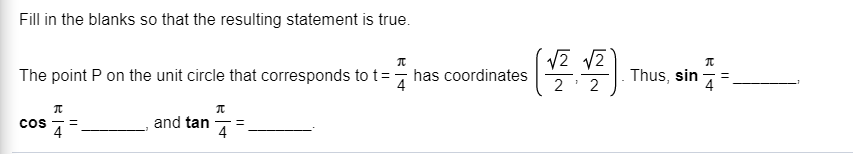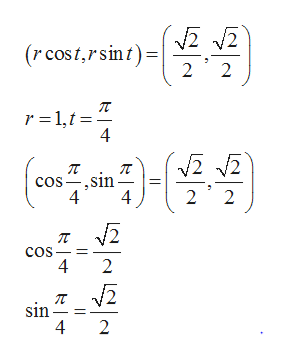# Fill in the blanks so that the resulting statement is true.The point P on the unit circle that corresponds to t has coordinatesThus, sin4=42 2and tanCOS4

Question
27 viewshelp_outlineImage TranscriptioncloseFill in the blanks so that the resulting statement is true. The point P on the unit circle that corresponds to t has coordinates Thus, sin 4 = 4 2 2 and tan COS 4 fullscreen
check_circle

star
star
star
star
star
1 Rating
Step 1

We know that  any point on the circle of radius r corresponds to t is ( rcost, rsint). Here r=1. Therefore, any point on unit circle corresponds t is (cost, sint), Substituting t=1 and comparing with the given point , we get required value of sine and cosine.help_outlineImage Transcriptionclose(rcost,rsint 2 2 r 1,t 4 cos-,sin 2 2 4 4 cos 4 2 sin 4 2 fullscreen
Step 2

Now, by dividing the value of sine by the value of cosi...

### Want to see the full answer?

See Solution

#### Want to see this answer and more?

Solutions are written by subject experts who are available 24/7. Questions are typically answered within 1 hour.*

See Solution
*Response times may vary by subject and question.
Tagged in
MathTrigonometry

### Trigonometric Ratios